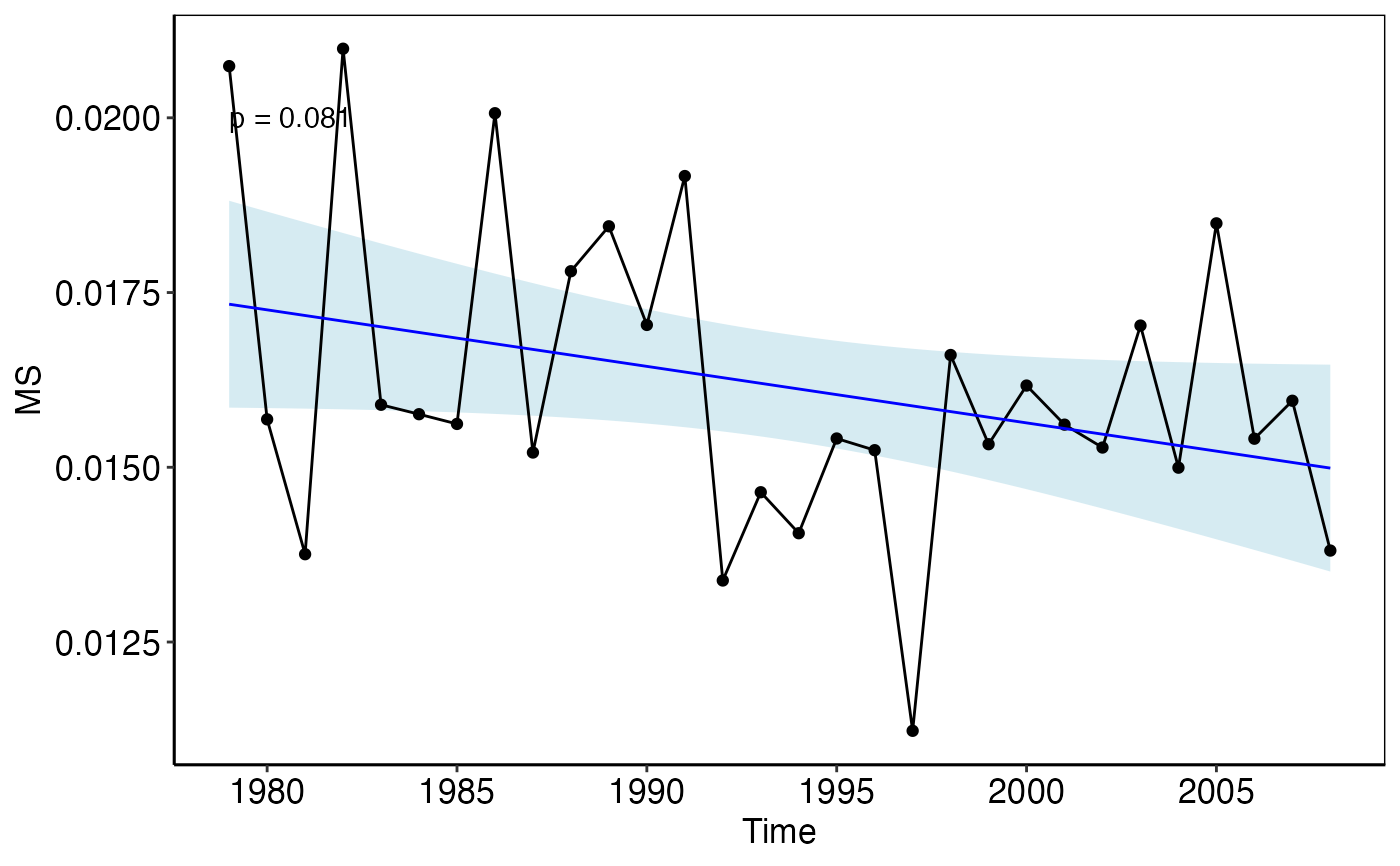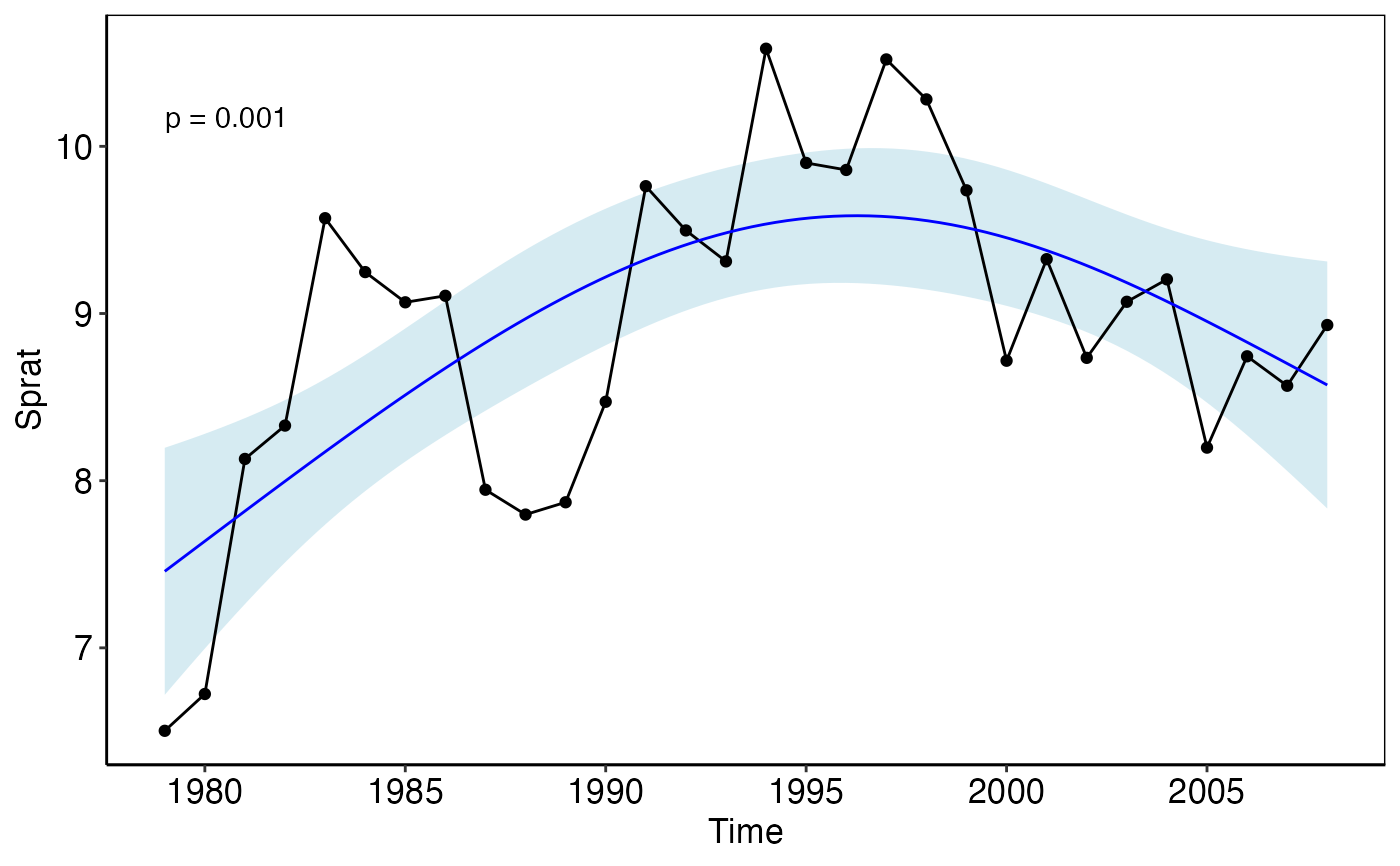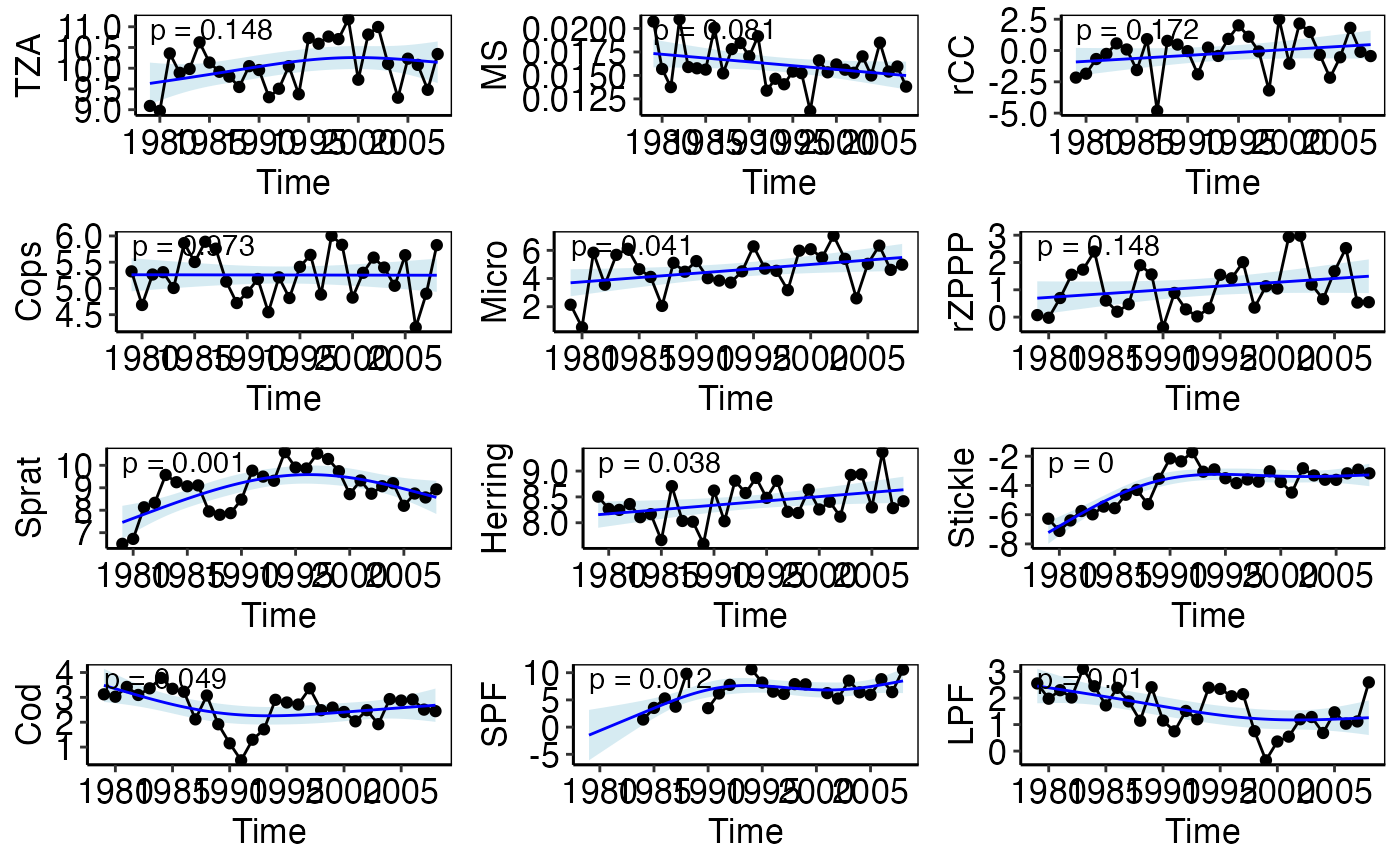plot_trend creates for each indicator (IND) in the input tibble a time series plot including the smoothed trend with 95% confidence interval and the corresponding p- value based on the IND ~ time GAM.

plot_trend(trend_tbl, pos_label = "topleft")

## Arguments

trend_tbl Output tibble from the model_trend function. Specifies the position of the annotation in the plot. Should be one of "topleft" (default), "topright", "bottomleft" or "bottomright". For more details see place_text.

## Value

The function returns a list of ggplot objects; one for each indicator.

model_trend that generates the model tibble for this function

## Examples

# Using the example data
trend_tbl <- model_trend_ex
pt <- plot_trend(trend_tbl)
# Show single plots using indicator names or indices
pt[]pt\$Sprat# Show all plots together
gridExtra::grid.arrange(grobs = pt)
#> Warning: Removed 5 row(s) containing missing values (geom_path).#> Warning: Removed 8 rows containing missing values (geom_point).